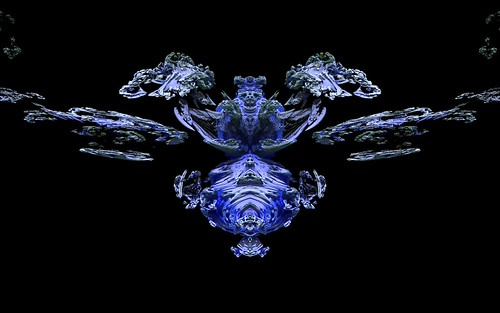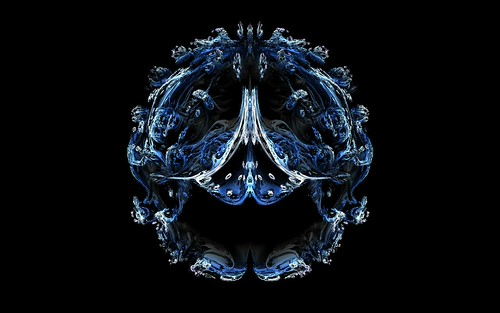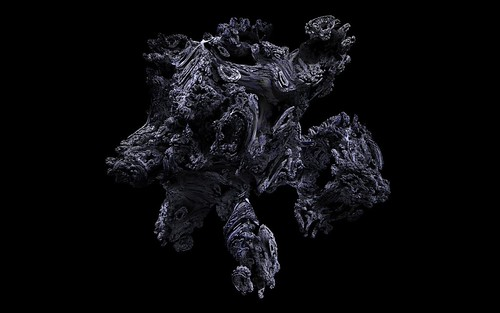# Mandelbulb and Juliabulb alternate formulas

The original Mandelbulb fractal began life as an attempt to find a 3D version of the 2D Mandelbulb formula z=z^2+c. Extending the z=z^2+c to z=z^p+c (p being any power exponent value) and using the newly invented Triplex Algebra gave rise to the Mandelbulb. Then other variations of the triplex algebra emerged which gave other varieties of Mandelbulbs.

So, to extend this further I have tested other formula variations on z=z^p+c to get some new Mandelbulbs (or maybe these should really be called Triplex Fractals as they use the triplex algebra, but are not real Mandelbulbs in the orginal sense).

Clicking the pictures will open gallery pages with more full sized example images.

z=z^p+z+c Mandelbulbsz=z^p+z+c Juliabulbsz=z^p-z+c Mandelbulbsz=z^p-z+c JuliabulbsThe above formula variations are now included in Visions Of Chaos.

Jason.